Question

Electrostatics

(a) Given that the magnetic field generated at the centre of an air-cored solenoid is given by B = µonI, where n is the number of turns per unit length, and I is the current, show that the self-inductance of a long solenoid of length I and area A is equal to µon²lA.

(b) A solenoid with I = 10 cm and diameter 1 cm is connected to a battery via a switch. The solenoid has 2000 turns per metre and is made of copper wire with resistivity 1.7 × 10-8 ohms m.Estimate the time taken after the switch is closed for the magnetic field to reach 90% of its maximum value. (Assume that the wires in the solenoid are packed as close together as they can be.)

(c) How would your answer to (b) change if the core of the solenoid were filled with iron with relative permeability of 5000?

(d) Consider the case where the battery has an EMF of 12 V and there is a resistor with R = 1000 2in series with the solenoid. Find the energy stored in the solenoid after the current has settled to a constant value for: (i) the air-cored solenoid, and (ii) the iron-cored solenoid.Verified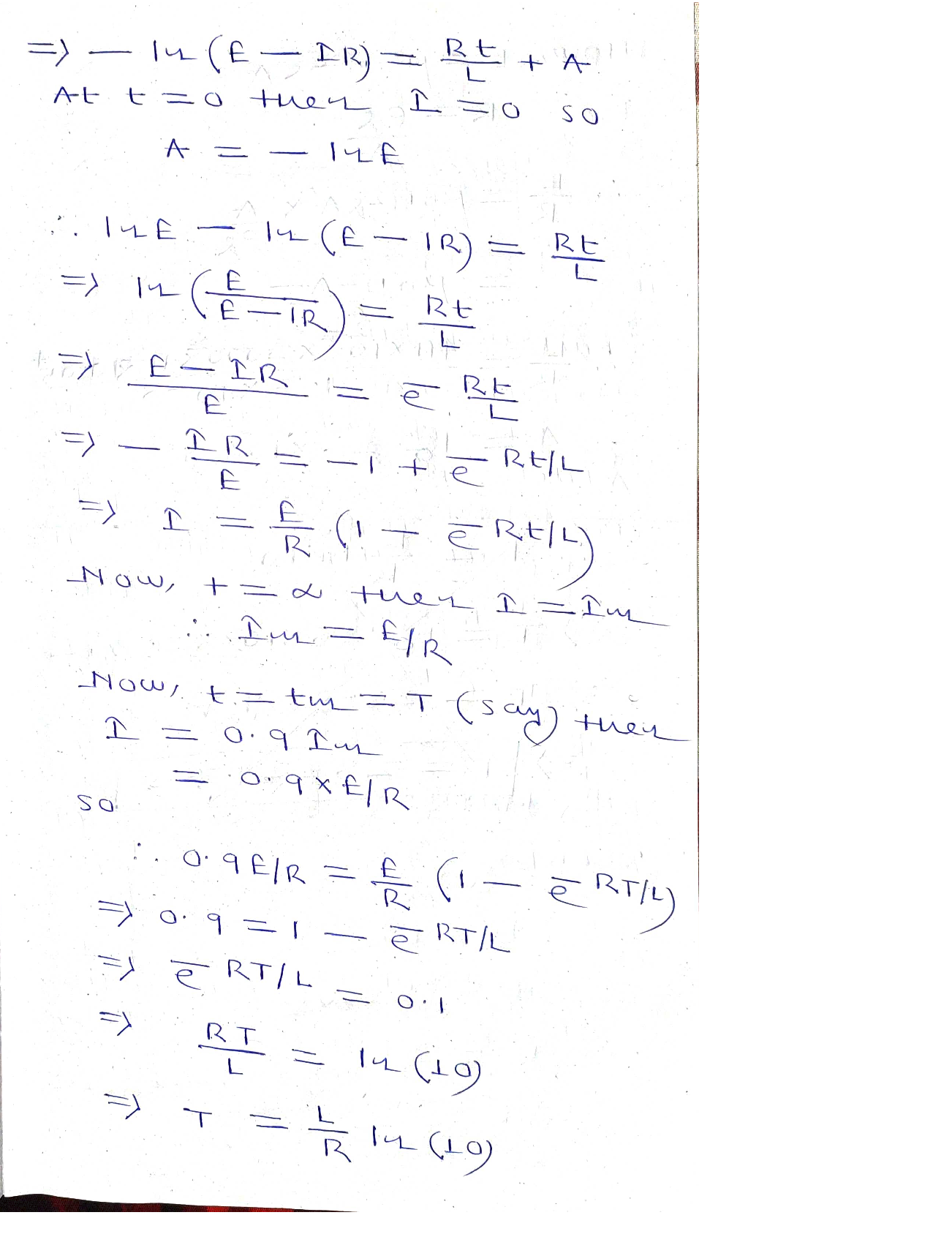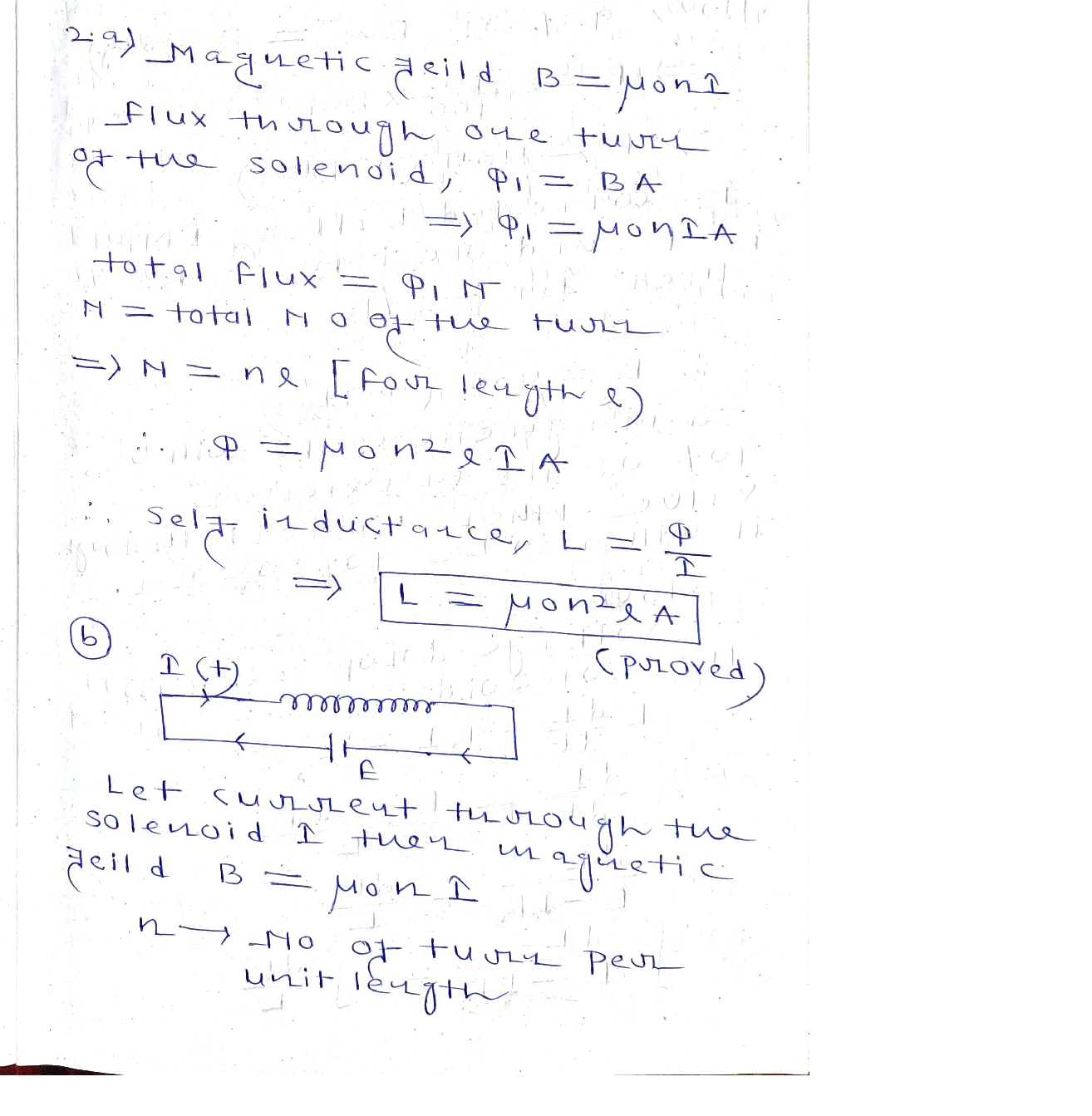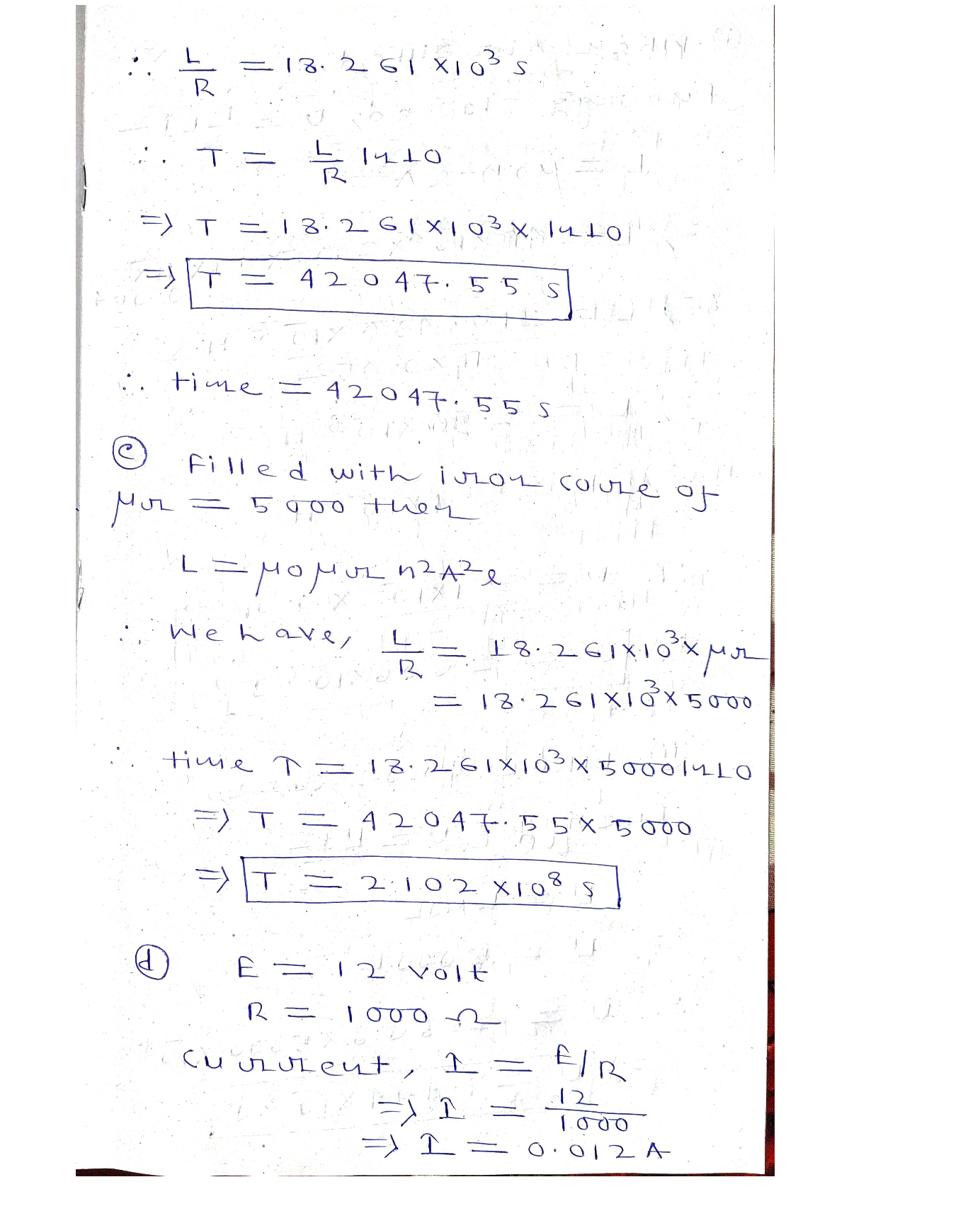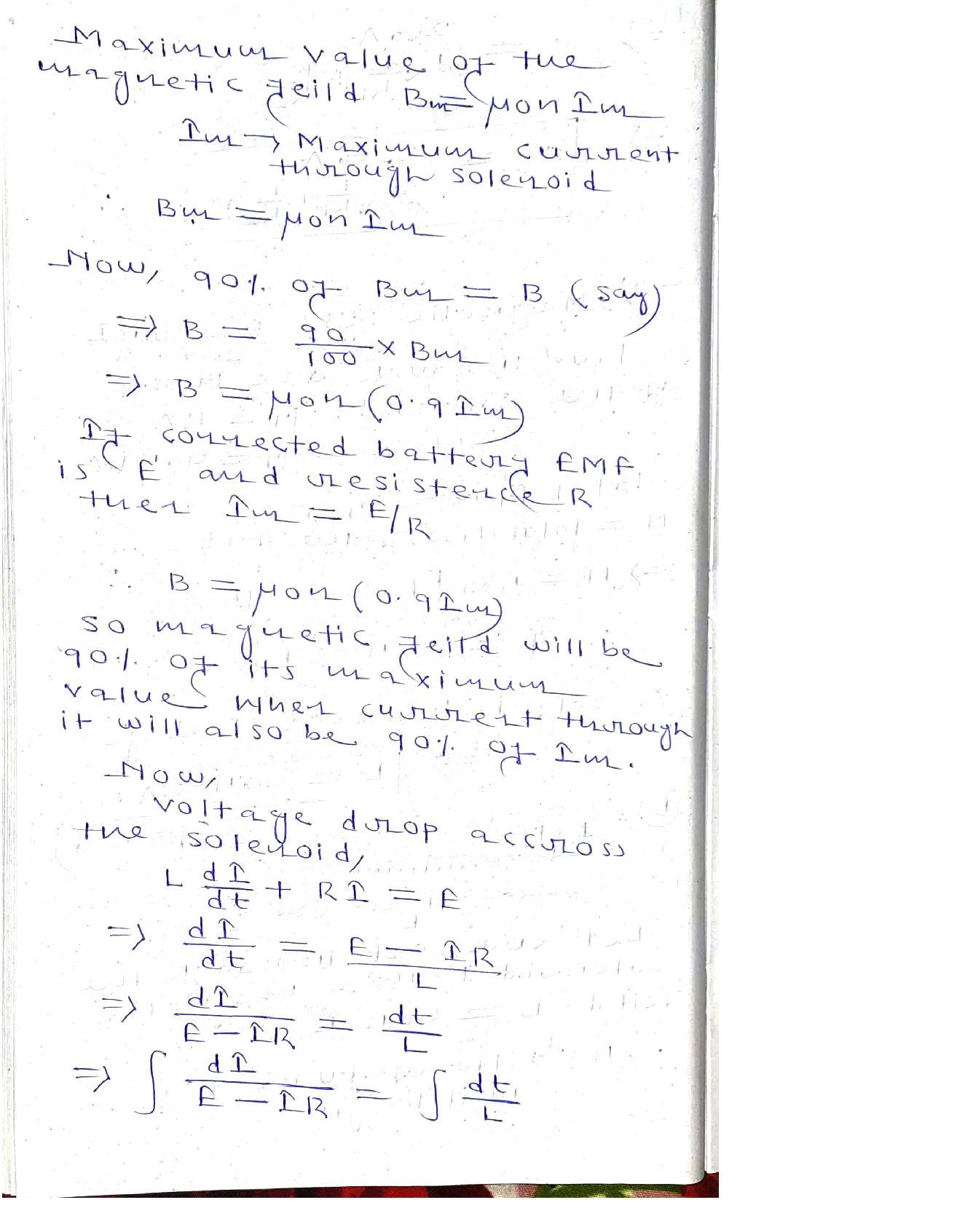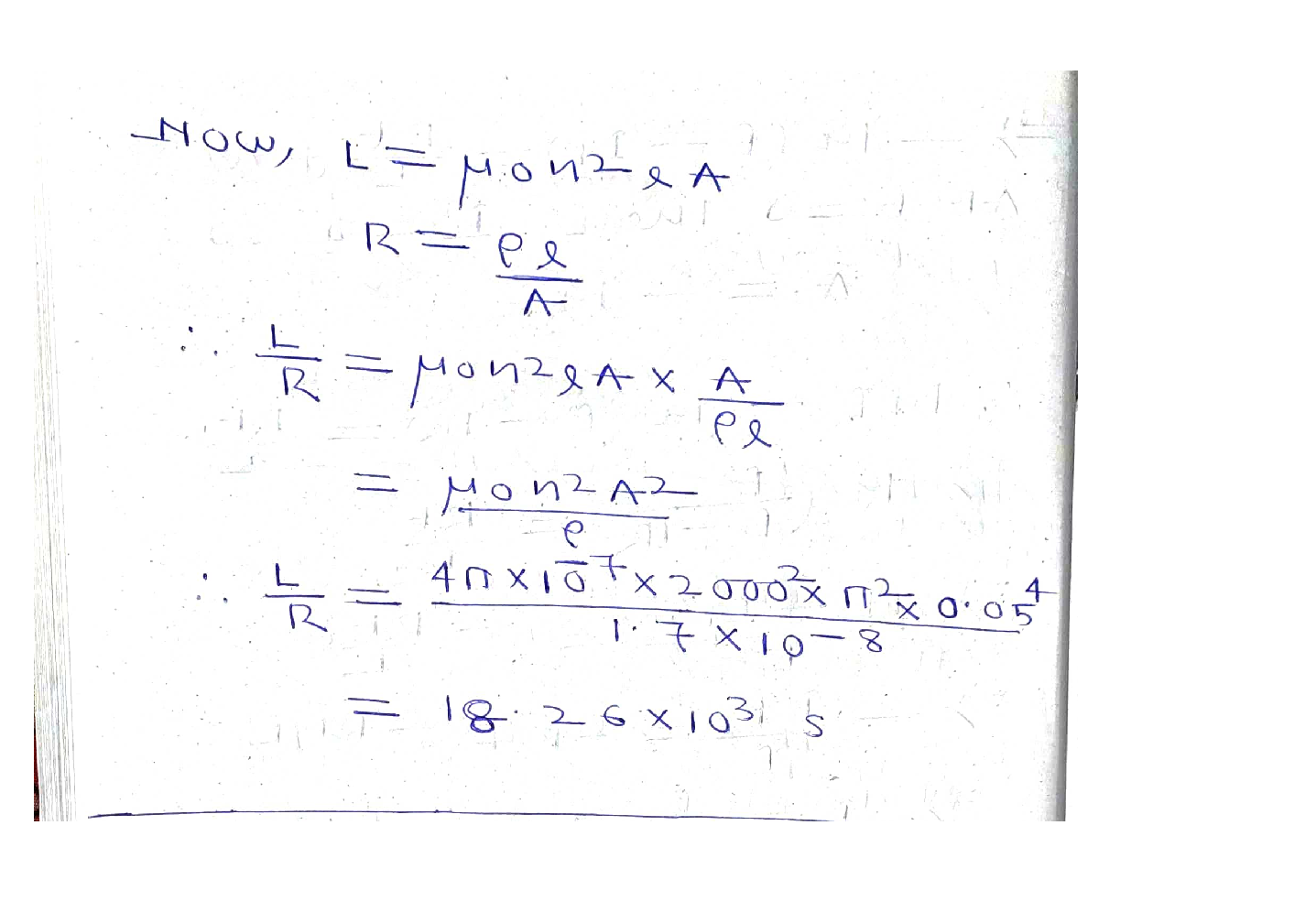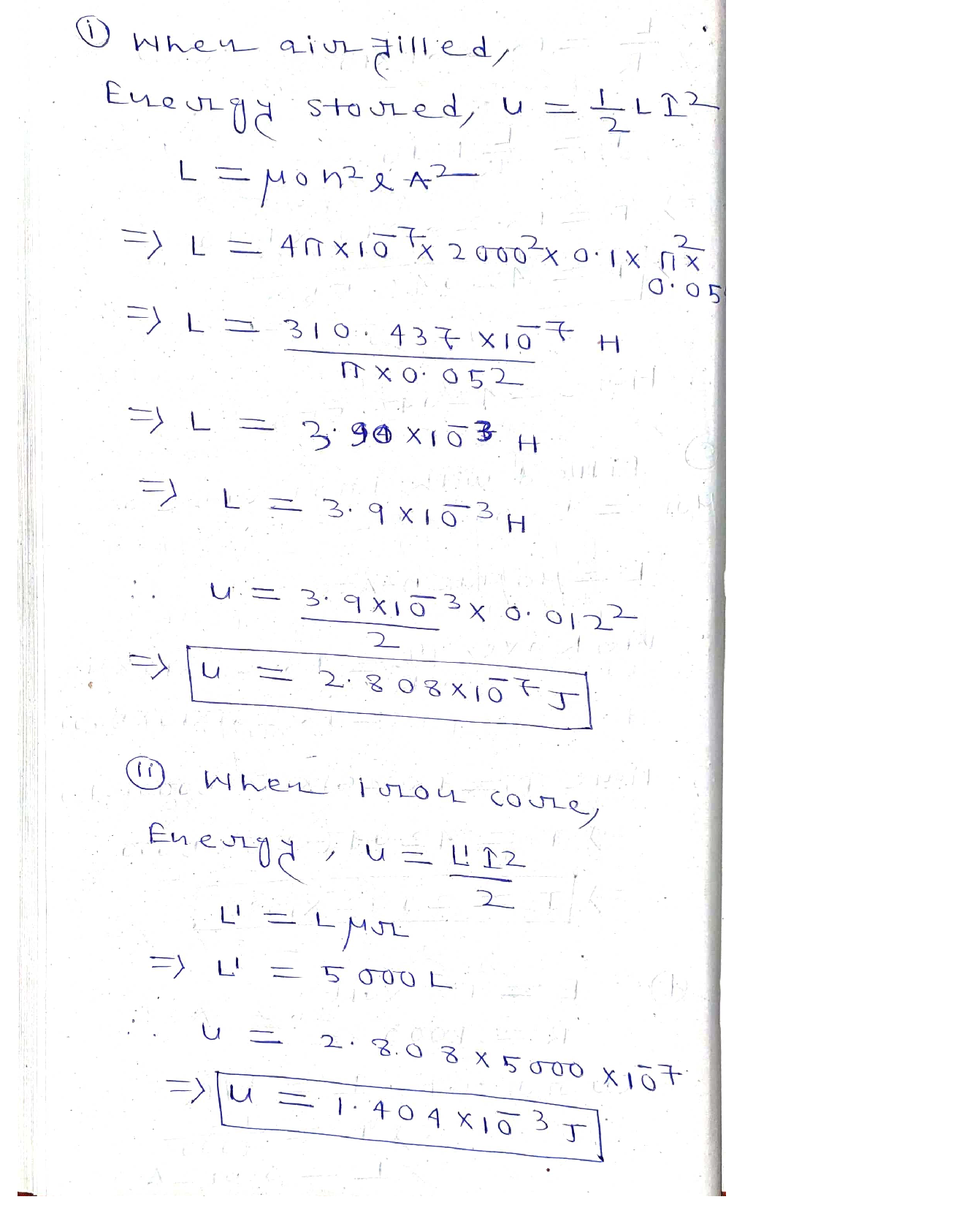### Question 45505Electrostatics

5. (20 points) When a clean surface of silver is irradiated with light of wavelength 230nm, the kinetic energy of the ejected electrons is measured to be 0.805 eV. Calculate the work function and the threshold frequency of silver.

### Question 45504Electrostatics

4. Consider a Copper metal shaped as an elongated parallelepiped. An electric field isapplied to this sample in the +y direction and the magnetic field is applied in the +zdirection, as schematically shown in the Figure below.
(a) (2 points) Indicate the direction (choose among +x, -x, +y, -y, +z, -z) in which the moving electrons are deflected/accumulated by the magnetic field.
(b) (2 points) What is the name of the force causing this deflection?
(d) (14 points) A Hall effect experiment is carried out at room temperature and the following results are obtained: RH = -0.55 x 10-1ºm³A's', o = 5.9 x 107 Q''m' Calculate the drift mobility and concentrations of conduction electrons in copper.
(c) (2 points) Indicate the direction (choose among +x, -x, +y, -y, +z, -z) of the Hallfield (the electric field set up by the deflected electrons).

### Question 45503Electrostatics

3. Consider a 1 kW AM radio transmitter at 700 kHz.
ts) Calculate the values of wavelength and energy for this photon.
ats) Calculate the number of photons emitted per second from the antenna.

### Question 45502Electrostatics

2. (20 points) The resistivity of pure gold at 0°C (273K) is measured to be 25 x 10-9 Q m.The temperature coefficient of resistance for Au is 1/233 K-'. Calculate the resistivity of gold at -40°C.

### Question 45501Electrostatics

1. The resistivity of aluminum (Al, Z=13) at 25 °C has been measured to be 2.72 x 10-8 Nm. Density is 2.70 g cm³ and atomic mass, Mat, is 27 g/mol.
Es) How many valence electrons does Al atom have?
(c) (5 points) Calculate the concentration (#/cm³ or #/m³) of conduction electrons.Provide any assumption used.
(d) (10 points) Calculate the drift mobility and mean free time between collisions for theconduction electrons in aluminum at 25 °C.
) What type of primary bonding do you expect Al to have?

### Question 43072Electrostatics

54. For the circuit in the figure below, four capacitors of capacitances 0.5 µF,1.5 µF, 2 µF and 4 µF are used. B is a bulb of unknown resistance R.
5 ma(a) Calculate the equivalent capacitance of the 4 capacitors.
(b) Knowing that the time constant of the circuit is 12 ms, calculate the resistance of the bulb.(5 mo
(c) At t = 0, an ideal battery delivering 12 V is connected between nodes a and b. Calculate how long after connecting the battery the current through the bulb reaches 1 mA.
(d) Calculate the energy stored on all capacitors when the currentthrough the bulb is 1 mA.
(e) Calculate the energy dissipated on the bulb and the total charge stored in the capacitors a long period of time after connecting the battery.

### Question 43071Electrostatics

Q lies3. A charge of q1at (a, 0) as shown below.Q lies at (-a, 0) and an identical charge of q2
(a) Find an expression for the electric potential at position (0, 6) (i.e.at any point along the y-axis) in terms of Q, a, b and the Coulomb constant, k. You should take the usual convention that electric potential is zero at infinity.а,[5 marks)
(b) Find an expression for the electric field at position (0, 6) (i.e. atany point along the y-axis) in terms of Q, a, b, and the Coulomb constant, k.[8 marks]
(c) For the case where Q = 2 nC and a =which must be done against the electric field to bring a charge of3 nC from infinity to position (0, 5) m.3 m, determine the work[4 marks]
(d) Now assume gravity acts downwards in the diagram (i.e. along the negative y direction). A charge of 3 nC placed at (0,5) m, initially at rest, is found to accelerate upwards at 2 m/s². Again assuming= 2 nC and a =3 m, determine the mass of the charge.[8 marks]

### Question 42871Electrostatics

Q15Consider the circuit below. R1 = 12 k2 and R2 = 8 k2 . The inductor has an inductance of 16 mH and at time zero has a current of 6 A. Calculate the energy stored in the inductor at t = 1.4 us, in units of uJ.

### Question 42870Electrostatics

Consider the circuit below. R1 = 5 k2 , and R2 = 3 k2. The capacitor has capacitance17 nF, and at time O it has charge 740 nC. Calculate the charge on the capacitor after 6.1us, in nC.

### Question 42869Electrostatics

Consider the circuit below. The odd numbered resistors have 6 ohms of resistance, and the even numbered resistors have 9 ohms of resistance. The battery outputs 79 V.Calculate the power output of the battery in this circuit, in W.

### Submit query

Getting answers to your urgent problems is simple. Submit your query in the given box and get answers Instantly.

### Submit a new QuerySuccess

Assignment is successfully created